# 指针和引用

comeweijjd枫长LH...大约 21 分钟

### # 1. *a和&a有什么区别（引用和指针有什么区别）？

``````int x = 10;
int *ptr = &x; // ptr 是一个指向 x 的指针
``````

``````int x = 10;
int &ref = x; // ref 是 x 的引用
``````

### # 2. 指针可以是 volatile 吗？

``````int volatile *ptr; // 指针指向一个 volatile 类型的整数
``````

``````int * volatile ptr; // 指针本身是 volatile 的
``````

``````int volatile * volatile ptr; // 指针本身和指向的整数都是 volatile 的
``````

`volatile` 关键字通常与硬件寄存器、中断服务例程或多线程编程等场景一起使用，以确保编译器不会对相关变量进行不必要的优化，从而导致潜在的问题。

### # 3. 指针常量和常量指针有什么区别？

``````const int *ptr;
``````

`ptr` 是一个指向常量整数的指针。通过这个指针，不能修改它所指向的整数的值。但可以让 `ptr` 指向其他整数对象。

``````int *const ptr = &x;
``````

`ptr` 是一个指向整数的指针常量。不能改变 `ptr` 的值，即它必须始终指向同一个整数对象（在这里是 `x`）。然而可以通过 `ptr` 修改它所指向的整数的值。

``````const int *const ptr = &x;
``````

### # 4. 什么情况用指针当参数，什么时候用引用，为什么？

• 当希望在函数内修改传递的参数时，使用引用是一种更直观和易读的方式。使用引用可以避免显式解引用和修改指针所指向的值。
• 当参数类型是类或结构时，使用引用可以避免复制成本。这对于大型对象或容器尤其重要，因为它们的复制可能会导致性能下降。
• 当你需要一个对象的别名，且这个别名在函数内部始终指向同一个对象时，使用引用。
• 当编写操作符重载函数时，通常使用引用，因为这可以提供更自然的语法和易用性。

• 当参数是可选的，即可以传递 `nullptr` 作为有效值时，使用指针。引用不能为 `nullptr`，而指针可以。
• 当需要在函数内部改变指针本身时，例如在链表或树的操作中，使用指针。
• 当需要显式地表示所有权和生命周期时，使用指针。指针可与智能指针（如 `std::unique_ptr``std::shared_ptr`）结合使用，以更清晰地表示资源所有权和生命周期。

### # 5. 从汇编层去解释一下引用？

C++ 代码：

``````#include <iostream>

void increment(int &x) {
x++;
}

int main() {
int a = 10;
increment(a);
std::cout << a << std::endl;
return 0;
}
``````

``````# increment 函数的调用
mov eax, DWORD PTR [rbp-4]    # 将变量 a 的内存地址放入 eax 寄存器
lea rdi, [rax]                # 将 eax 寄存器的值（a 的内存地址）放入 rdi 寄存器，rdi 是 increment 函数的第一个参数
call increment                # 调用 increment 函数
...
``````

### # 6. 数组名和指针有什么区别？

``````int arr;     // arr 是一个整数数组，包含 5 个元素
int *ptr = arr; // ptr 是一个整数指针，指向 arr 的首元素

arr = ptr;      // 错误！不能修改数组名的值
ptr = &arr;  // 正确，可以修改指针的值
``````

### # 7. 野指针是什么？

``````int *ptr; // 未初始化的指针
int value = *ptr; // 未知行为，因为指针指向不确定的内存区域
``````

``````int *ptr = new int(10);
delete ptr; // 释放内存
int value = *ptr; // 未知行为，因为指针指向的内存已被释放
``````

``````int *ptr;

void func() {
int x = 10;
ptr = &x;
} // x 的生命周期在函数退出时结束

func();
int value = *ptr; // 未知行为，因为指针指向的局部变量已超出生命周期
``````

### # 8. 如何检测内存泄漏？

• 使用智能指针：C++11引入了智能指针（例如`std::unique_ptr``std::shared_ptr`），这些指针可以自动管理内存。当智能指针的生命周期结束时，它们会自动释放所指向的内存。

• 重载new和delete：可以重载全局或类特定的`new``delete`操作符，以便跟踪分配和释放的内存。通过这种方式发现没有被正确释放的内存。

• 使用内存泄漏检测工具：有许多现成的工具可以帮助你检测C++程序中的内存泄漏。这些工具有助于识别和定位内存泄漏，以便你可以修复它们。一些流行的内存泄漏检测工具包括：

• Valgrind：这是一个功能强大的Linux下的内存泄漏检测工具。它可以检测出许多内存泄漏和其他内存相关的问题。
• Visual Leak Detector：这是一个用于Microsoft Visual Studio的内存泄漏检测插件。它可以帮助你在Windows平台上检测内存泄漏。
• 静态代码分析：使用静态代码分析工具（例如Clang-Tidy、Cppcheck等）可以在编译时自动检测潜在的内存泄漏和其他问题。

• 代码审查和测试：定期进行代码审查和编写测试用例可以识别和预防内存泄漏。

### # 9. 如何避免“野指针”？

``````int* ptr = nullptr;
``````

``````delete ptr;
ptr = nullptr;
``````

### # 10. 常引用有什么作用？

``````const int& const_ref = some_int_variable;
``````

``````void func(const std::vector<int>& vec) {
// 使用vec进行操作，但不会修改它
}
``````

``````class MyClass {
public:
void someFunction() {
// 非const版本
}

void someFunction() const {
// const版本
}
};
``````

### # 11. C++中的指针参数传递和引用参数传递？

• 指针传递意味着将指针的副本传递给函数。因此，函数接收到的是指向原始数据的指针。
• 指针传递允许你修改所指向的数据，但不允许修改指针本身。因为传递的是指针的副本，所以在函数内部修改指针不会影响到原始指针。
• 指针可以为`nullptr`，这可能导致运行时错误。在使用指针之前，需要检查其有效性。
• 语法较为繁琐，需要使用指针操作符`*``->`来访问和修改数据。
``````void pointer_pass(int* ptr) {
if (ptr) {
*ptr = 10; // 修改所指向的数据
}
}
``````

• 引用传递意味着将原始数据的别名（引用）传递给函数。因此，函数接收到的是原始数据的引用，而不是副本。
• 引用传递允许直接修改原始数据，无需额外的操作符。
• 引用必须在创建时初始化，且不能重新绑定到其他对象。因此，引用通常比指针更安全。
• 语法更简洁，无需使用特殊操作符。引用的使用与普通变量相似。
``````void reference_pass(int& ref) {
ref = 10; // 直接修改原始数据
}
``````

• 指针传递通过传递指向原始数据的指针副本来实现。它允许修改所指向的数据，但不能修改指针本身。指针传递需要额外的操作符，并且可能引入`nullptr`错误。
• 引用传递通过传递原始数据的别名（引用）来实现。它允许直接修改原始数据，并具有更简洁的语法。引用传递通常比指针传递更安全。

### # 13. 指针和引用之间如何转换？

``````int x = 10;
int &ref = x;       // ref 是 x 的引用
int *ptr = &ref;    // ptr 是指向 x 的指针
``````

``````int y = 20;
int *ptr2 = &y;     // ptr2 是指向 y 的指针
int &ref2 = *ptr2;  // ref2 是 y 的引用
``````

### # 14. 智能指针有哪些以及有什么作用？

`std::unique_ptr`

``std::unique_ptr`是一种独占式智能指针，表示对动态分配内存的唯一所有权。`unique_ptr`在作用域结束时自动释放它所管理的内存。由于它不能被复制，但可以通过`std::move`进行转移，因此确保了一个对象在任何时刻最多只有一个`unique_ptr`指向它。

``````#include <memory>

void func() {
std::unique_ptr<int> uptr(new int(42)); // uptr 独占指向动态分配的整数
} // uptr 超出作用域，自动释放内存
``````

`std::shared_ptr`

`std::shared_ptr`是一种共享式智能指针，允许多个`shared_ptr`共享对同一个对象的所有权。`shared_ptr`使用引用计数机制来跟踪有多少个`shared_ptr`指向同一个对象。当最后一个指向该对象的`shared_ptr`超出作用域或被销毁时，对象的内存将被自动释放。

``````#include <memory>

void func() {
std::shared_ptr<int> sptr1(new int(42));
{
std::shared_ptr<int> sptr2 = sptr1; // sptr1 和 sptr2 共享指向同一个整数
} // sptr2 超出作用域，但由于 sptr1 仍然存在，所以不释放内存
} // sptr1 超出作用域，引用计数为0，自动释放内存
``````

`std::weak_ptr`

`std::weak_ptr`是一种弱引用智能指针，它不对所指向的对象的内存进行管理。`weak_ptr`通常与`shared_ptr`一起使用，用于避免循环引用（导致内存泄漏）的问题。`weak_ptr`可以从`shared_ptr`或另一个`weak_ptr`创建。要访问`weak_ptr`指向的对象，需要先将其提升为`shared_ptr`

``````#include <memory>

std::shared_ptr<int> sptr(new int(42));
std::weak_ptr<int> wptr = sptr;

if (auto locked = wptr.lock()) { // 提升为 shared_ptr
// 使用 locked 访问对象
} else {
// 对象已被释放
}
``````

### # 15. shared_ptr出现循环引用怎么解决？

`shared_ptr` 是 C++ 中的一种智能指针，它能自动管理引用计数，当引用计数为零时，智能指针所指向的对象会被自动销毁。但是，当出现循环引用时，智能指针可能会导致内存泄漏。

``````#include <iostream>
#include <memory>

class B; // 前向声明

class A {
public:
std::shared_ptr<B> b_ptr;
~A() { std::cout << "A destroyed" << std::endl; }
};

class B {
public:
std::weak_ptr<A> a_ptr; // 使用 weak_ptr 替换 shared_ptr
~B() { std::cout << "B destroyed" << std::endl; }
};

int main() {
{
std::shared_ptr<A> a = std::make_shared<A>();
std::shared_ptr<B> b = std::make_shared<B>();
a->b_ptr = b;
b->a_ptr = a;
}
// 当离开作用域时，A 和 B 实例将被正确销毁，避免了内存泄漏
return 0;
}
``````

### # 16. 使用智能指针管理内存资源，RAII？

`std::unique_ptr`: 一种独占所有权的智能指针，同一时间只能有一个 `unique_ptr` 指向给定的对象。当 `unique_ptr` 离开作用域或被销毁时，它所指向的对象也会被自动销毁。这种智能指针适用于单一所有权的场景。

``````#include <iostream>
#include <memory>

class MyClass {
public:
MyClass() { std::cout << "MyClass constructor" << std::endl; }
~MyClass() { std::cout << "MyClass destructor" << std::endl; }
};

int main() {
{
std::unique_ptr<MyClass> ptr = std::make_unique<MyClass>();
// 当离开作用域时，MyClass 实例将被自动销毁
}
std::cout << "MyClass instance destroyed" << std::endl;
return 0;
}
``````

`std::shared_ptr`: 允许多个 `shared_ptr` 实例共享同一个对象的所有权。引用计数器跟踪指向该对象的智能指针数量，当引用计数为零时，对象将被自动销毁。这种智能指针适用于多个所有者共享资源的场景。

`std::weak_ptr`: 一种弱引用智能指针，它不会增加引用计数。它通常与 `shared_ptr` 一起使用，以避免循环引用导致的内存泄漏。

### # 17. 手写实现智能指针类？

``````#include <iostream>

template <typename T>
class SmartPointer {
public:
// 构造函数
explicit SmartPointer(T* ptr = nullptr) : _ptr(ptr), _count(ptr ? new int(1) : nullptr) {}

// 拷贝构造函数
SmartPointer(const SmartPointer<T>& other) : _ptr(other._ptr), _count(other._count) {
if (_count) {
++(*_count);
}
}

// 赋值操作符
SmartPointer<T>& operator=(const SmartPointer<T>& other) {
if (this != &other) {
release();
_ptr = other._ptr;
_count = other._count;
if (_count) {
++(*_count);
}
}
return *this;
}

// 析构函数
~SmartPointer() {
release();
}

// 重载解引用操作符
T& operator*() const {
return *_ptr;
}

// 重载箭头操作符
T* operator->() const {
return _ptr;
}

// 获取引用计数
int use_count() const {
return _count ? *_count : 0;
}

private:
// 释放资源
void release() {
if (_count && --(*_count) == 0) {
delete _ptr;
delete _count;
}
}

T* _ptr;
int* _count;
};

int main() {
SmartPointer<int> sp1(new int(10));
std::cout << "sp1 use_count: " << sp1.use_count() << std::endl;

SmartPointer<int> sp2(sp1);
std::cout << "sp1 use_count: " << sp1.use_count() << std::endl;
std::cout << "sp2 use_count: " << sp2.use_count() << std::endl;

return 0;
}

``````

•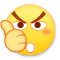0
•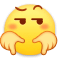0
•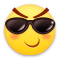0
•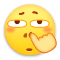0
•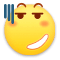0
•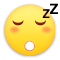0

• 按正序
• 按倒序
• 按热度